# Hexagon

Divide a regular hexagon into lines into nine completely identical parts; none of them must be in a mirror image (individual parts can only be rotated arbitrarily).

n =  0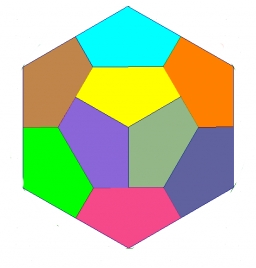Did you find an error or inaccuracy? Feel free to write us. Thank you!#### You need to know the following knowledge to solve this word math problem:

We encourage you to watch this tutorial video on this math problem:

## Related math problems and questions:

• Hexagon = 8 parts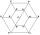Divide the regular hexagon into eight equal parts.
• Divide in ratioLine segment AB 12 cm long divide in a ratio of 5: 3. How long are the individual parts?
• Hexagon rotationA regular hexagon of side 6 cm is rotated through 60° along a line passing through its longest diagonal. What is the volume of the figure thus generated?
• Divide an isosceles triangleHow to divide an isosceles triangle into two parts with equal contents perpendicular to the axis of symmetry (into a trapezoid and a triangle)?
• Dividing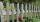Divide the three-line segment 13 cm, 26 cm, and 19.5 cm long for parts so that the individual pieces were equally long and longest. How long will the individual parts, and how many will it?
• HexagonThere is regular hexagon ABCDEF. If area of the triangle ABC is 22, what is area of the hexagon ABCDEF? I do not know how to solve it simply....
• Line segment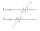Line segment AB is 8 cm long. Divide it in a ratio of 2: 3.
• MO Z8–I–6 2018In the KLMN trapeze, KL has a 40 cm base and an MN of 16 cm. Point P lies on the KL line so that the NP segment divides the trapezoid into two parts with the same area. Find the length of the KP line.
• Line segmentCut a line segment of 15 cm into two line segments so that their lengths are in ratio 2:1. What length will each have?
• Chocholate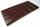Table of chocolate is divided into squares on its surface. Lengthwise has 15 squares and widthwise 19 squares. We must chocolate broke into individual squares. How many times have we broke it to get only individual squares? It is not permitted to break se
• Hexagon ABCDEFIn the regular hexagon ABCDEF, the diagonal AE has a length 8cm. Calculate the circumference and the hexagon area.
• Coordinates hexagon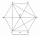The regular hexagon ABCDEF is given. Point A has coordinates [1; 3], and point D has coordinates [4; 7]. Calculate the sum of the coordinates of the center of its described circle.
• Hexagon areaThe center of the regular hexagon is 21 cm away from its side. Calculate the hexagon side and its area.
• MO - trianglesOn the AB and AC sides of the triangle ABC lies successive points E and F, on segment EF lie point D. The EF and BC lines are parallel and is true this ratio FD:DE = AE:EB = 2:1. The area of ABC triangle is 27 hectares and line segments EF, AD, and DB seg
• Trapezoid MO-5-Z8ABCD is a trapezoid that lime segment CE is divided into a triangle and parallelogram, as shown. Point F is the midpoint of CE, DF line passes through the center of the segment BE, and the area of the triangle CDE is 3 cm2. Determine the area of the trape
• Rotatable tower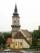Rotatable tower situated in the city center has ground shape of a regular polygon. If the tower is rotated by 18° around its centerpiece it looks from the side same. Your task is to calculate at least how many vertices can have a ground plan view of the t
• LinesHow many lines can be draw with 8 points, if three points lie on one line and the other any three points do not lie on the same line?